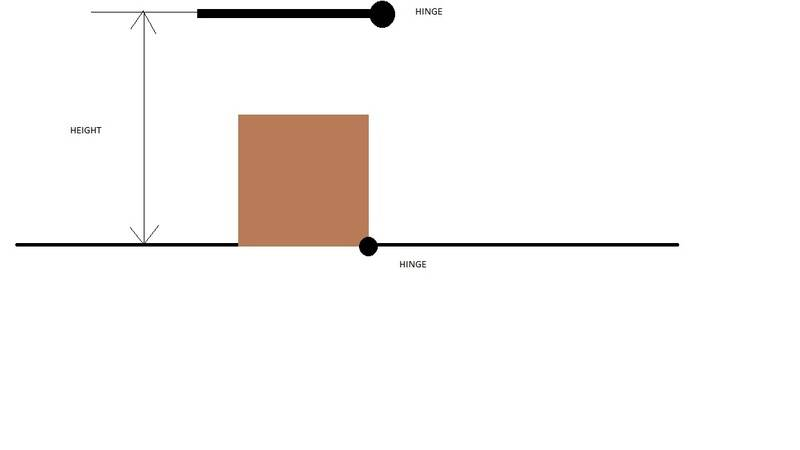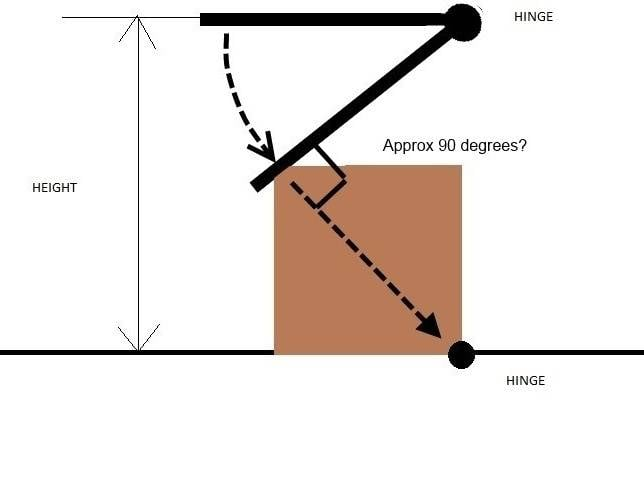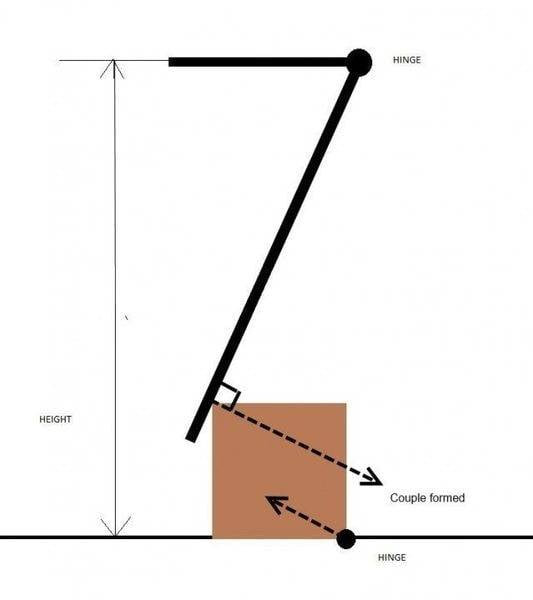# Problem with classical mechanics

• TonyEsposito
In summary, the cube will rise if the height of the rod is greater than the distance the cube is from the ground when the rod falls.f

#### TonyEsposito

Hi guys i have a problem and I'm not able to solve it. I have a cube lying on the ground that is hinged on a side in contact with the floor. Right above the hinge there is a rod hinged (at the start time the rod is horizzontal) and it is long enough to touch the cube when it fall...how much the height must be in order to the rod to be able to rotate the cube after the collision?I don't believe you have enough information to solve the problem.

One problem I see is that at impact the line of force could be towards the pivot point of the block. See diagram..In that case there would be no torque acting on the block and it wouldn't rotate. The hinge point for the rod might have to be moved a lot higher and the rod made a lot longer to change this angle significantly.One approach would be to calculate the Potential Energy (PE) of the rod in the up position. That would be turned into rotational KE as the rod falls. Then you would need to do some calculations to work out how much energy might be transferred to the block. I'm not quite sure how to tackle that because I think it would be difficult to apply conservation of angular momentum.

Ok, thanks for the hints. I used to see this problem in an exam, i thinked about using some energy conservation principle but i have no idea how to proceed ;)

Problem is energy is only conserved for an elastic collision. Momentum is conserved for both elastic an inelastic collisions.

yeah, i forgot to say that the problem say that the collision is elastic

If you have a copy of the problem statement best post that word for word?

It's in the italian language, I'm going to translate it as best as i can: "There is a cube of mass M and side length a and it is lying on a plane where an edge of the cube is hinged to that. Vertically above the hinge, at an height h from the plane there is a rod hinged, the rod have a constant linear density p, the rod's length is long enough to be able to touch the opposite edge of the cube. The rod is left falling from an horizzontal position and it collide elastically with the cube. Calculate for which values of h the cube is going to rise from the plane after the collision (warning: think before writing anything and only then write the answer, do not be surprised by the banality of the problem)."
That's all :p